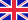•# Introduction to Partial Differential Equations

Autor: Gerald B. Folland

The second edition of Introduction to Partial Differential Equations, which originally appeared in the Princeton series Mathematical Notes, serves as a text for mathematics students at the intermediate graduate level. The goal is to acquaint readers with... Viac o knihe

Na objednávku, dodanie 2-4 týždne

104.10 €

bežná cena: 118.30 €

## O knihe

The second edition of Introduction to Partial Differential Equations, which originally appeared in the Princeton series Mathematical Notes, serves as a text for mathematics students at the intermediate graduate level. The goal is to acquaint readers with the fundamental classical results of partial differential equations and to guide them into some aspects of the modern theory to the point where they will be equipped to read advanced treatises and research papers. This book includes many more exercises than the first edition, offers a new chapter on pseudodifferential operators, and contains additional material throughout.

The first five chapters of the book deal with classical theory: first-order equations, local existence theorems, and an extensive discussion of the fundamental differential equations of mathematical physics. The techniques of modern analysis, such as distributions and Hilbert spaces, are used wherever appropriate to illuminate these long-studied topics. The last three chapters introduce the modern theory: Sobolev spaces, elliptic boundary value problems, and pseudodifferential operators.

• Vydavateľstvo: Princeton University Press
• Rok vydania: 1995
• Formát: Hardback
• Rozmer: 235 x 157 mm
• Jazyk: Anglický jazyk
• ISBN: 9780691043616

Generuje redakčný systém BUXUS CMS spoločnosti ui42.